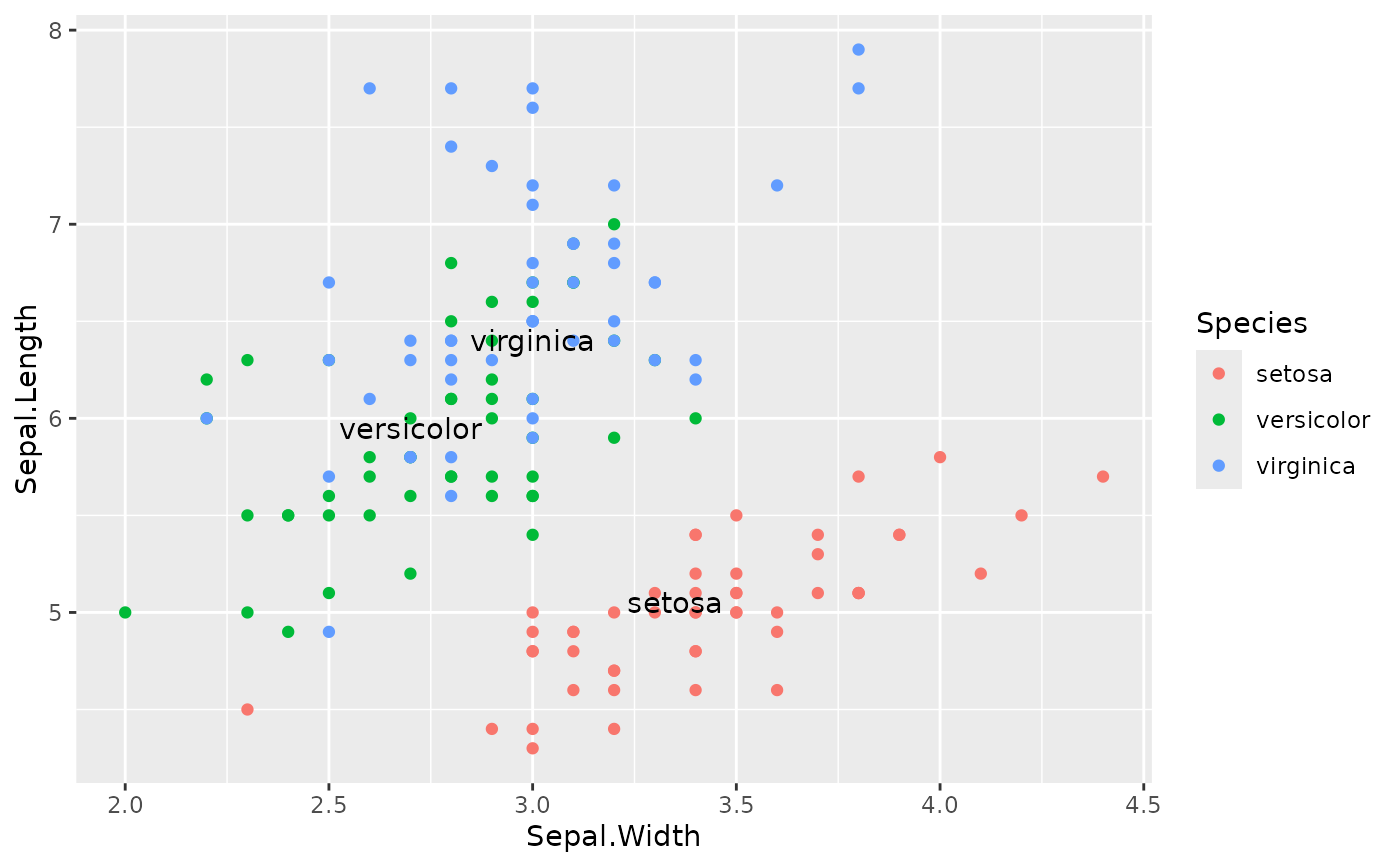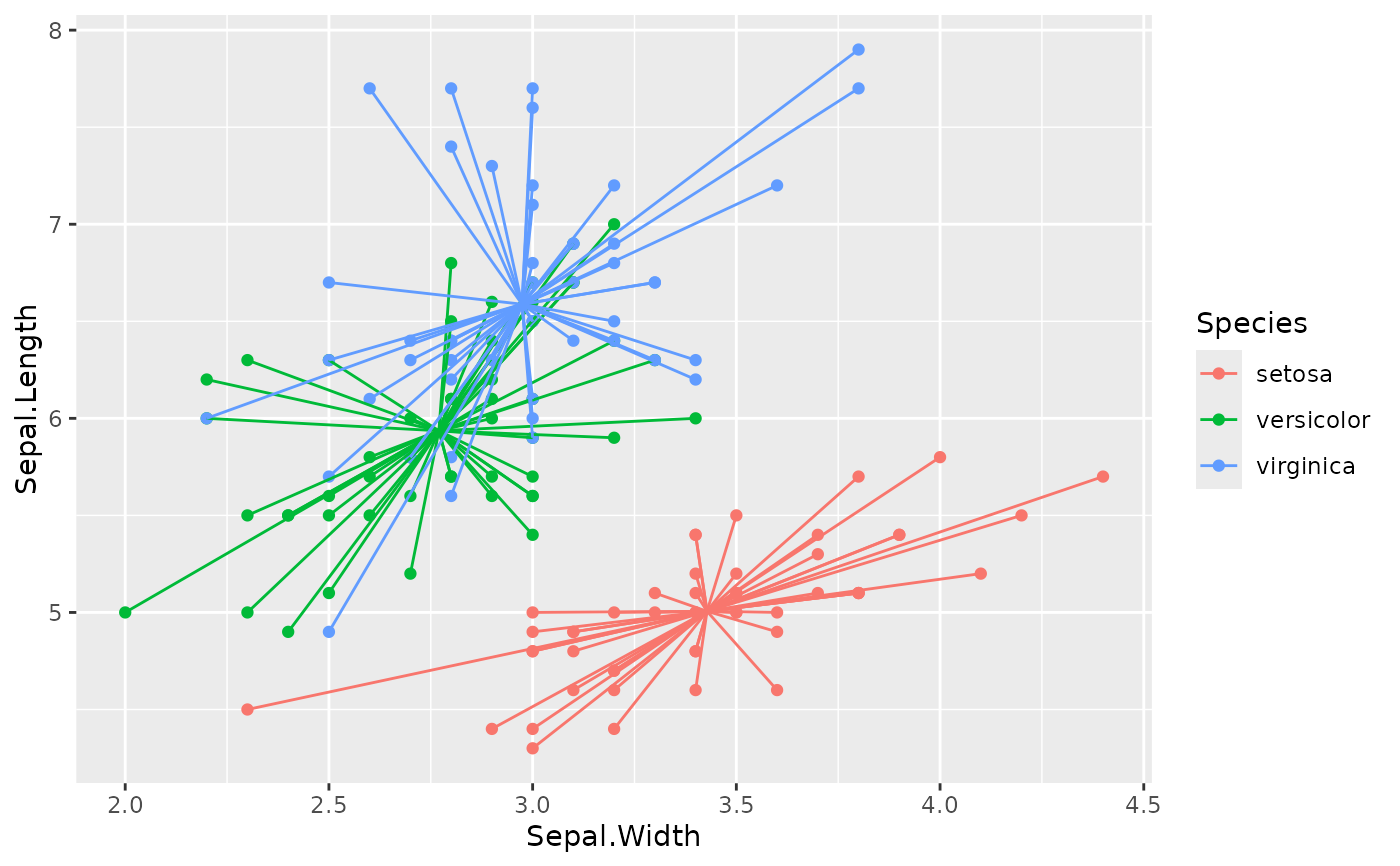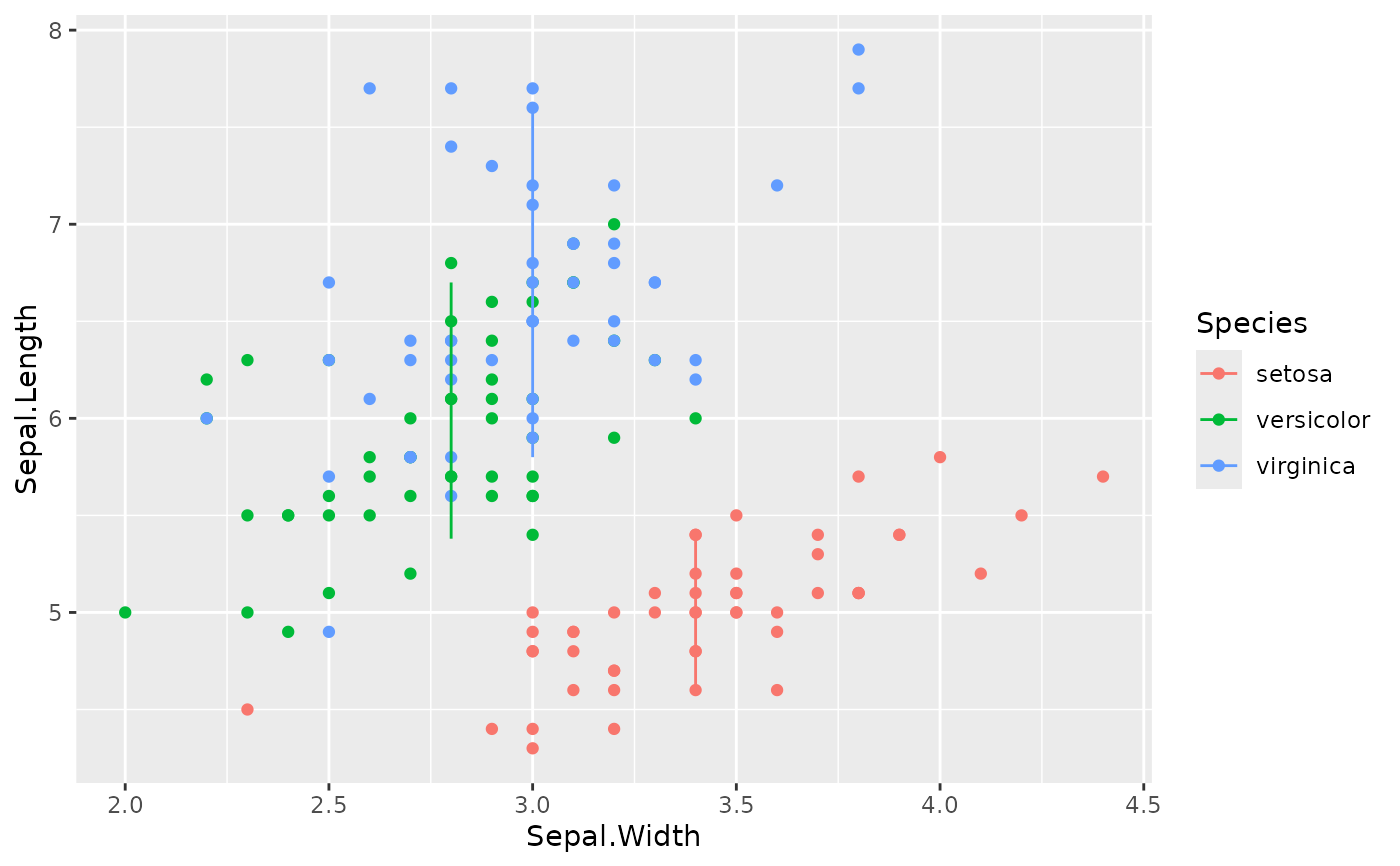The function xy stat applies a function to the x- and y-coordinates of a layers positions by group. The stat_centroid() and stat_midpoint() functions are convenience wrappers for calculating centroids and midpoints. stat_funxy() by default leaves the data as-is, but can be supplied functions and arguments.

## Usage

stat_funxy(
mapping = NULL,
data = NULL,
geom = "point",
position = "identity",
...,
funx = force,
funy = force,
argx = list(),
argy = list(),
crop_other = TRUE,
show.legend = NA,
inherit.aes = TRUE
)

stat_centroid(
...,
funx = mean,
funy = mean,
argx = list(na.rm = TRUE),
argy = list(na.rm = TRUE)
)

stat_midpoint(..., argx = list(na.rm = TRUE), argy = list(na.rm = TRUE))

## Arguments

mapping

Set of aesthetic mappings created by aes(). If specified and inherit.aes = TRUE (the default), it is combined with the default mapping at the top level of the plot. You must supply mapping if there is no plot mapping.

data

The data to be displayed in this layer. There are three options:

If NULL, the default, the data is inherited from the plot data as specified in the call to ggplot().

A data.frame, or other object, will override the plot data. All objects will be fortified to produce a data frame. See fortify() for which variables will be created.

A function will be called with a single argument, the plot data. The return value must be a data.frame, and will be used as the layer data. A function can be created from a formula (e.g. ~ head(.x, 10)).

geom

The geometric object to use to display the data, either as a ggproto Geom subclass or as a string naming the geom stripped of the geom_ prefix (e.g. "point" rather than "geom_point")

position

Position adjustment, either as a string naming the adjustment (e.g. "jitter" to use position_jitter), or the result of a call to a position adjustment function. Use the latter if you need to change the settings of the adjustment.

...

Other arguments passed on to layer(). These are often aesthetics, used to set an aesthetic to a fixed value, like colour = "red" or size = 3. They may also be parameters to the paired geom/stat.

funx, funy

A function to call on the layer's x and y positions respectively.

argx, argy

A named list containing arguments to the funx, and funy function calls.

crop_other

A logical of length one; whether the other data should be fitted to the length of x and y (default: TRUE). Useful to set to FALSE when funx or funy calculate summaries of length one that need to be recycled.

show.legend

logical. Should this layer be included in the legends? NA, the default, includes if any aesthetics are mapped. FALSE never includes, and TRUE always includes. It can also be a named logical vector to finely select the aesthetics to display.

inherit.aes

If FALSE, overrides the default aesthetics, rather than combining with them. This is most useful for helper functions that define both data and aesthetics and shouldn't inherit behaviour from the default plot specification, e.g. borders().

## Value

A StatFunxy ggproto object, that can be added to a plot.

## Details

This statistic only makes a minimal attempt at ensuring that the results from calling both functions are of equal length. Results of length 1 are recycled to match the longest length result.

## Examples

p <- ggplot(iris, aes(Sepal.Width, Sepal.Length, colour = Species))

# Labelling group midpoints
p + geom_point() +
stat_midpoint(aes(label = Species, group = Species),
geom = "text", colour = "black")# Drawing segments to centroids
p + geom_point() +
stat_centroid(aes(xend = Sepal.Width, yend = Sepal.Length),
geom = "segment", crop_other = FALSE)# Drawing intervals
ggplot(iris, aes(Sepal.Width, Sepal.Length, colour = Species)) +
geom_point() +
stat_funxy(geom = "path",
funx = median, funy = quantile,
argy = list(probs = c(0.1, 0.9)))